Courses

# Very Short Answer Type Questions - Quadratic Equations Class 10 Notes | EduRev

## Class 10 : Very Short Answer Type Questions - Quadratic Equations Class 10 Notes | EduRev

The document Very Short Answer Type Questions - Quadratic Equations Class 10 Notes | EduRev is a part of the Class 10 Course Class 10 Mathematics by VP Classes.
All you need of Class 10 at this link: Class 10

Q1. If the roots of the quadratic equation − ax2 + bx + c = 0 are equal then show that b2 = 4ac.

Sol. ∵ For equal roots, we have
b2 − 4ac =0
∴ b2 = 4ac

Q2. Find the value of ‘k’ for which the quadratic equation kx2 − 5x + k = 0 have real roots.

Sol. Comparing kx2 − 5x + k = 0 with ax2 + bx + c = 0, we have:

a = k
b = − 5
c = k

∴ b2 − 4ac =(− 5)2 − 4 (k) (k)
= 25 − 4k2

For equal roots, b2 − 4ac = 0
∴ 25 − 4k2 = 0
⇒ 4k2 = 25

⇒ k= 25/4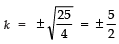∴    b2 = 4ac

Q3. If 2 is a root of the equation x2 + kx + 12 = 0 and the equation x2 + kx + q = 0 has equal roots, find the value of q.

Sol. Since, 2 is a root of x2 + kx + 12 = 0
∴ (2)2 + k(2) + 12 = 0
or 4 + 2k + 12 = 0
⇒ 2k = −16 or  k = − 8
Roots of  x2 + kx + q = 0 are equal
∴ k2 − 4(1) (q) = 0 or  k2 − 4q = 0
But k = −8, so (−8)2 = 4q  or  q = 16

Q4. If − 4 is a root of the quadratic equation x2 + px − 4 = 0 and x2 + px + k = 0 has equal roots, find the value of k.

Sol. ∵ (–4) is a root of x+ px − 4 = 0
∴ (− 4)2 + p (− 4) = 0
⇒ 16 − 4p − 4= 0
⇒ 4p = 12 or p = 3
Now, x2 + px + k = 0
⇒ x2 + 3x + k = 0    [∵ p = 3]
Now, a = 1, b = 3 and c = + k
∴ b2 − 4ac = (3)2 − 4 (1) (k)
= 9 − 4k
For equal roots, b2 − 4ac = 0

⇒ 9 − 4k =0 ⇒ 4k = 9

⇒ k = 9/4

Q5. If one root of the quadratic equation 2x2 − 3x + p = 0 is 3, find the other root of the quadratic equation. Also, find the value of p.

Sol. We have: 2x2 − 3x + p = 0 ...(1)
∴ a = 2, b = − 3 and c = p

Since, the sum of the roots = -b/a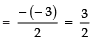∵ One of the roots = 3
∴ The other root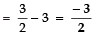Now, substituting x = 3 in (1), we get

2 (3)2 − 3 (3) + p =0
⇒ 18 − 9 + p = 0
⇒ 9 + p = 0
⇒ p  = − 9

Q6. If one of the roots of x2 + px − 4 = 0 is − 4 then find the product of its roots and the value of p.

Sol. If − 4 is a root of the quadratic equation,

x2 + px − 4=0
∴ (− 4)2 + (− 4) (p) − 4 = 0
⇒ 16 − 4p − 4 = 0
⇒ 12 − 4p = 0
⇒ p  = 3

Now, in ax2 + bx + c = 0, the product of the roots = c/a

∴ Product of the roots in x2 − px − 4= 0

= -4/1 = -4

Q7. For what value of k, does the given equation have real and equal roots? (k + 1) x2 − 2 (k − 1) x + 1 = 0.

Sol. Comparing the given equation with ax2 + bx + c = 0, we have:

a = k + 1
b = − 2 (k − 1)
c = 1

For equal roots, b2 − 4ac = 0

∴ [− 2 (k − 1)]− 4 (k + 1) (1) = 0
⇒ 4 (k − 1) − 4 (k + 1) = 0
⇒ 4 (k + 1 − 2k) − 4k − 4 = 0
⇒ 4k + 4 − 8k − 4k − 4 = 0
⇒ 4k − 12k = 0
⇒ 4k (k − 3) = 0
⇒ k = 0 or k = 3

x2 − 2ax + (a2 − b2) = 0

Sol. Comparing x2 − 2ax + (a2 − b2) = 0, with ax2 + bx + c = 0, we have:

a = 1, b = − 2a, c = a2 − b2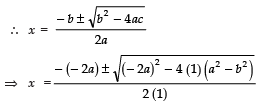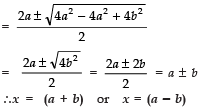∴ x =( a + b) or x = (a − b)

Q9. If one of the roots of the quadratic equation 2x2 + kx − 6 = 0 is 2, find the value of k. Also, find the other root.

Sol. Given equation:

2x2 + kx − 6= 0
one root = 2
Substituting x = 2 in 2x+ kx − 6 = 0
We have:

2 (2)2 + k (2) − 6= 0
⇒ 8 + 2k − 6= 0
⇒ 2k + 2 = 0  ⇒ k = − 1
∴ 2x2 + kx − 6 = 0  ⇒ 2x2 − x − 6 = 0

Sum of the roots  = -b/a = 1/2
∴ other root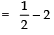= 3/2

Q10. Determine the value of k for which the quadratic equation 4x2 − 4kx + 1 = 0 has equal roots.

Sol. We have:

4x2 − 4kx + 1 = 0
Comparing with ax2 + bx + c = 0,
we have
a = 4, b = − 4k and c = 1
∴ b2 − 4ac =(− 4k)2 − 4 (4k) (1)
= 16k2 − 16

For equal roots

b2 − 4ac = 0

∴ 16k2 − 16 = 0

⇒ 16k2 = 16 ⇒ k2 = 1
⇒ k = ± 1

Q11. For what value of k, does the quadratic equation x2 − kx + 4 = 0 have equal roots?

Sol. Comparing x2 − kx + 4 = 0 with ax2 + bx + c = 0, we get
a = 1
b = − k
c = 4

∴ b2 − 4ac =(− k)2 − 4 (1) (4) = k2 − 16

For equal roots,

b2 − 4ac =0
⇒ k2 − 16 = 0
⇒ k2 = 16
⇒ k = ±  4

Q12. What is the nature of roots of the quadratic equation 4x2 − 12x + 9 = 0?

Sol. Comparing 4x2 − 12x + 9 = 0 with ax2 + bx + c = 0 we get

a = 4
b = − 12
c = 9

∴ b2 − 4ac =(− 12)2 − 4 (4) (9)
= 144 − 144 = 0

Since b2 − 4ac = 0

∴ The roots are real and equal.

Q13. Write the value of k for which the quadratic equation x2 − kx + 9 = 0 has equal roots.

Sol. Comparing x− kx + 9 = 0 with ax2 + bx + c = 0, we get

a = 1
b = − k
c = 9

∴ b2 − 4ac = (− k)2 − 4 (1) (9)
= k2 − 36

For equal roots, b2 − 4ac = 0
⇒ k2 − 36 = 0  ⇒ k2 = 36
⇒ k = ±  6

Q14. For what value of k are the roots of the quadratic equation 3x2 + 2kx + 27 = 0 real and equal?

Sol. Comparing 3x2 + 2 kx + 27 = 0 with ax2 + bx + c = 0, we have:

a = 3
b = 2k
c = 27

∴ b2 − 4ac = (2k)2 − 4 (3) (27)
= 4k2 − (12 × 27)

For the roots to be real and equal

b2 − 4ac = 0
⇒ 4k2 − (12 × 17) = 0
⇒ 4k2 = 12 × 27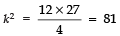⇒ k = ± 9

Q15. For what value of k are the roots of the quadratic equation kx2 + 4x + 1 = 0 equal and real?

Sol. Comparing kx2 + 4x + 1 = 0, with ax2 + bx + c = 0, we get

a = k
b = 4
c = 1

∴ b2 − 4ac = (4)− 4 (k) (1)
= 16 − 4k

For equal and real roots, we have

b2 − 4ac =0
⇒ 16 − 4k = 0
⇒ 4k = 16

⇒ k = 16/4 = 4

Q16. For what value of k does (k − 12) x2 + 2 (k − 12) x + 2 = 0 have equal roots?

Sol. Comparing (k − 12) x2 + 2 (k − 12) x + 2 = 0 with ax2 + bx + c = 0, we have:

a = (k − 12)
b = 2 (k − 12)
c = 2

∴ b2 − 4ac = [2 (k − 12)]2 − 4 (k − 12) (2)
= 4 (k − 12)2 − 8 (k − 12)
= 4 (k − 12) [k − 12 − 2]
= 4 (k − 12) (k − 14)

For equal roots,

b2 − 4ac =0
⇒ 4 (k − 12) [k − 14] = 0
⇒ Either 4 (k − 12) = 0 ⇒ k = 12

or k − 14 = 0 ⇒ k = 14

But k = 12 makes k − 12 = 0 which is not required

∴ k ≠ 12
⇒ k = 14

Q17. For what value of k does the equation 9x2 + 3kx + 4 = 0 has equal roots?

Sol. Comparing 9x2 + 3kx + 4 = 0 with ax2 + bx + c = 0, we get

a = 9
b = 3k
c = 4

∴ b2 − 4ac =(3k)2 − 4 (9) (4)
= 9k2 − 144
For equal roots,
b2 − 4ac = 0
⇒ 9k2 − 144 = 0
⇒9 k2 = 144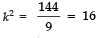⇒ k = ±  4

Offer running on EduRev: Apply code STAYHOME200 to get INR 200 off on our premium plan EduRev Infinity!

132 docs

,

,

,

,

,

,

,

,

,

,

,

,

,

,

,

,

,

,

,

,

,

;